# IBPS PO Prelims Quantitative Aptitude Questions 2021 (Day-01)

Dear Aspirants, Our IBPS Guide team is providing new series of Quantitative Aptitude Questions for IBPS PO Prelims 2021 so the aspirants can practice it on a daily basis. These questions are framed by our skilled experts after understanding your needs thoroughly. Aspirants can practice these new series questions daily to familiarize with the exact exam pattern and make your preparation effective.

Start Quiz

Approximation

Directions (1-5): What approximate value should come in place of question mark in the following questions?

1)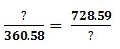A.613

B.513

C.469

D.552

E.507

2)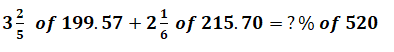A.201

B.210

C.250

D.235

E.221

3)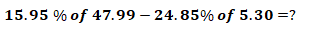A.7.95

B.6.43

C.8.58

D.4.54

E.3.65

4)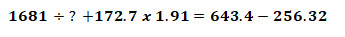A.63

B.56

C.50

D.41

E.51

5)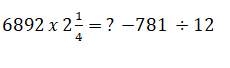A.15572

B.13788

C.13478

D.12557

E.11254

Table chart

Directions (06-10): Study the following information carefully and answer the questions given below.

The following table gives the information about the number of different mobiles sold by a shopkeeper over the year.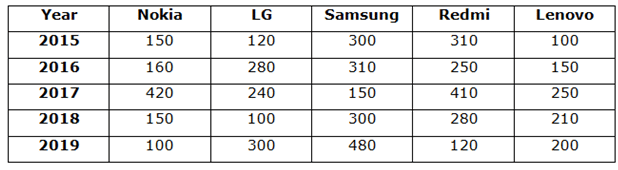6) What is the percentage increase in the sales of Samsung in 2019 compared to that of in 2015?

A.40%

B.50%

C.20%

D.48%

E.60%

7) What is the respective ratio of the Nokia mobile sold in 2017 and 2019 together and Redmi mobile sold in 2015 and 2016 together?

A.13: 14

B.14: 13

C.5: 7

D.7: 5

E.None of these

8) Find difference between the total number of all model mobiles sold by the shopkeeper in 2019 and the total number of all the model mobiles sold in 2016?

A.25

B.40

C.50

D.48

E.None of these

9) The total number of LG mobiles sold over the years is how much less than the total number of Redmi mobiles sold over the years?

A.120

B.330

C.200

D.299

E.None of these

10) The total number of Lenovo mobiles sold in 2016 and 2017 together is approximately what percent of the total number of Samsung mobiles sold in 2018 and 2019 together?

A.24%

B.17%

C.51%

D.15%

E.35%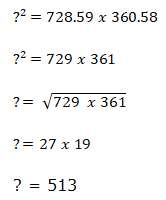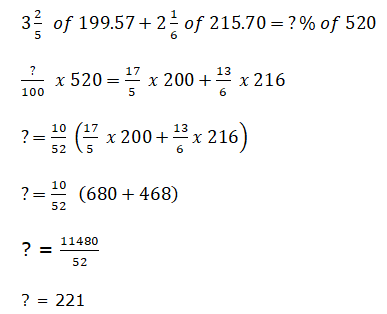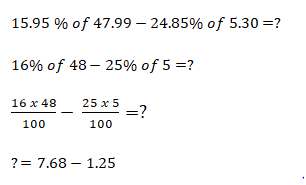? = 6.43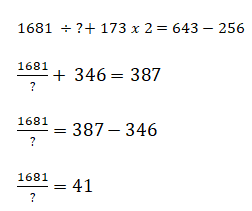? = 41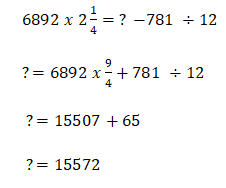Sales of Samsung in 2019 = 480

Sales of Samsung in 2015 = 300

Required % = (480 – 300)/300 * 100 = 60%

Nokia mobiles sold in 2017 and 2019 together = 420 + 100 = 520

Redmi mobiles sold in 2015 and 2016 together = 310 + 250 = 560

Required ratio = 520: 560 = 13: 14

Total number of all the model mobiles sold in 2019 = 100 + 300 + 480 + 120 + 200 = 1200

Total number of all the model mobiles sold in 2016 = 160 + 280 + 310 + 250 + 150 = 1150

Required difference = 1200 – 1150 = 50

Total number of LG mobiles sold over the years = 120 + 280 + 240 + 100 + 300

= 1040

Total number of Redmi mobiles sold over the years = 310 + 250 + 410 + 280 + 120 = 1370

Required difference = 1370 – 1040 = 330

Total number of Lenovo mobiles sold in 2016 and 2017 together = 150 + 250

= 400

Total number of Samsung mobiles sold in 2018 and 2019 together = 300 + 480

= 780

Required % = (400/780) * 100 = 51%